SLOS417D October   2003  – November 2015

PRODUCTION DATA.

1. Features
2. Applications
3. Description
4. Device Comparison Table
5. Pin Configuration and Functions
6. Specifications
7. Parameter Measurement Information
8. Detailed Description
1. 8.1 Overview
2. 8.2 Functional Block Diagram
3. 8.3 Feature Description
4. 8.4 Device Functional Modes
9. Application and Implementation
1. 9.1 Application Information
2. 9.2 Typical Applications
1. 9.2.1 TPA200110D1 With Differential Input
2. 9.2.2 TPA20010D1 With Differential Input and Input Capacitors
3. 9.2.3 TPA20010D1 with Single-Ended Input
10. 10Power Supply Recommendations
11. 11Layout
1. 11.1 Layout Guidelines
2. 11.2 Board Layout
3. 11.3 Layout Example
12. 12Device and Documentation Support
13. 13Mechanical, Packaging, and Orderable Information

• YZF|9

## 6 Specifications

### 6.1 Absolute Maximum Ratings

Over operating free-air temperature range (unless otherwise noted)(1)
MIN MAX UNIT
VDD Supply voltage In active mode –0.3 6 V
In SHUTDOWN mode
VI Input voltage –0.3 7 V
Continuous total power dissipation –0.3 VDD + 0.3 V
TA Operating free-air temperature See Dissipation Ratings °C
TJ Operating junction temperature –40 85 °C
Lead temperature 1,6 mm (1/16 inch) from case for 10 seconds YZF 260 °C
YEF 235 °C
Tstg Storage temperature –65 150 °C
(1) Stresses beyond those listed under Absolute Maximum Ratings may cause permanent damage to the device. These are stress ratings only, which do not imply functional operation of the device at these or any other conditions beyond those indicated under Recommended Operating Conditions. Exposure to absolute-maximum-rated conditions for extended periods may affect device reliability.

### 6.2 ESD Ratings

VALUE UNIT
V(ESD) Electrostatic discharge Human-body model (HBM), per ANSI/ESDA/JEDEC JS-001(1) ±1500 V
Charged-device model (CDM), per JEDEC specification JESD22-C101(2) ±1500
(1) JEDEC document JEP155 states that 500-V HBM allows safe manufacturing with a standard ESD control process.
(2) JEDEC document JEP157 states that 250-V CDM allows safe manufacturing with a standard ESD control process.

### 6.3 Recommended Operating Conditions

MIN NOM MAX UNIT
VDD Supply voltage 2.5 5.5 V
VIH High-level input voltage SHUTDOWN 1.3 VDD V
VIL Low-level input voltage SHUTDOWN 0 0.35 V
RI Input resistor Gain ≤ 20 V/V (26 dB) 15
VIC Common mode input voltage range VDD = 2.5 V, 5.5 V, CMRR ≤ –49 dB 0.5 VDD – 0.8 V
TA Operating free-air temperature –40 85 °C

### 6.4 Thermal Information

THERMAL METRIC(1) TPA2010D1 UNIT
YZF (DSBGA)
9 PINS
RθJA Junction-to-ambient thermal resistance 100.3 °C/W
RθJC(top) Junction-to-case (top) thermal resistance 0.7 °C/W
RθJB Junction-to-board thermal resistance 24.7 °C/W
ψJT Junction-to-top characterization parameter 3.5 °C/W
ψJB Junction-to-board characterization parameter 24.7 °C/W
RθJC(bot) Junction-to-case (bottom) thermal resistance n/a °C/W

### 6.5 Electrical Characteristics

TA = 25°C (unless otherwise noted)
PARAMETER TEST CONDITIONS MIN TYP MAX UNIT
|VOS| Output offset voltage
(measured differentially)
VI = 0 V, AV = 2 V/V, VDD = 2.5 V to 5.5 V 1 25 mV
PSRR Power supply rejection ratio VDD = 2.5 V to 5.5 V –75 –55 dB
CMRR Common mode rejection ratio VDD = 2.5 V to 5.5 V, VIC = VDD / 2 to 0.5 V,
VIC = VDD / 2 to VDD – 0.8 V
–68 –49 dB
|IIH| High-level input current VDD = 5.5 V, VI = 5.8 V 100 µA
|IIL| Low-level input current VDD = 5.5 V, VI = –0.3 V 5 µA
I(Q) Quiescent current VDD = 5.5 V, no load 3.4 4.9 mA
VDD = 3.6 V, no load 2.8
VDD = 2.5 V, no load 2.2 3.2
I(SD) Shutdown current V(SHUTDOWN) = 0.35 V, VDD = 2.5 V to 5.5 V 0.5 2 µA
rDS(on) Static drain-source on-state
resistance
VDD = 2.5 V 700
VDD = 3.6 V 500
VDD = 5.5 V 400
Output impedance in SHUTDOWN V(SHUTDOWN) = 0.35 V >1
f(sw) Switching frequency VDD = 2.5 V to 5.5 V 200 250 300 kHz
Gain VDD = 2.5 V to 5.5 V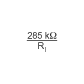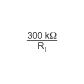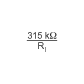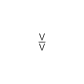Resistance from shutdown to GND 300

### 6.6 Operating Characteristics

TA = 25°C, Gain = 2 V/V, RL = 8 Ω (unless otherwise noted)
PARAMETER TEST CONDITIONS MIN TYP MAX UNIT
PO Output power THD + N = 10%, f = 1 kHz, RL = 4 Ω VDD = 5 V 2.5 W
VDD = 3.6 V 1.3
VDD = 2.5 V 0.52
THD + N = 1%, f = 1 kHz, RL = 4 Ω VDD = 5 V 2.08 W
VDD = 3.6 V 1.06
VDD = 2.5 V 0.42
THD + N = 10%, f = 1 kHz, RL = 8 Ω VDD = 5 V 1.45 W
VDD = 3.6 V 0.73
VDD = 2.5 V 0.33
THD + N = 1%, f = 1 kHz, RL = 8 Ω VDD = 5 V 1.19 W
VDD = 3.6 V 0.59
VDD = 2.5 V 0.26
THD+N Total harmonic distortion plus
noise
VDD = 5 V, PO = 1 W, RL = 8 Ω, f = 1 kHz 0.18%
VDD = 3.6 V, PO = 0.5 W, RL = 8 Ω, f = 1 kHz 0.19%
VDD = 2.5 V, PO = 200 mW, RL = 8 Ω, f = 1 kHz 0.20%
kSVR Supply ripple rejection ratio VDD = 3.6 V, Inputs ac-grounded
with Ci = 2 µF
f = 217 Hz,
V(RIPPLE) = 200 mVpp
–67 dB
SNR Signal-to-noise ratio VDD = 5 V, PO = 1 W, RL = 8 Ω 97 dB
Vn Output voltage noise VDD = 3.6 V, f = 20 Hz to 20 kHz,
Inputs ac-grounded with Ci = 2 µF
No weighting 48 µVRMS
A weighting 36
CMRR Common mode rejection ratio VDD = 3.6 V, VIC = 1 Vpp f = 217 Hz –63 dB
ZI Input impedance 142 150 158
Start-up time from shutdown VDD = 3.6 V 1 ms

### 6.7 Dissipation Ratings

PACKAGE DERATING FACTOR(1) TA ≤ 25°C
POWER RATING
TA = 70°C
POWER RATING
TA = 85°C
POWER RATING
YZF 7.8 mW/°C 780 mW 429 mW 312 mW
(1) Derating factor measure with High K board.

### 6.8 Typical Characteristics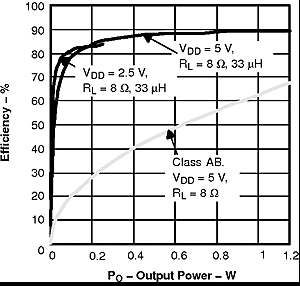Figure 1. Efficiency versus Output PowerFigure 3. Power Dissipation versus Output Power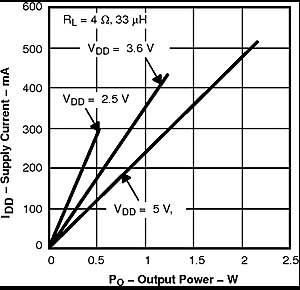Figure 5. Supply Current versus Output Power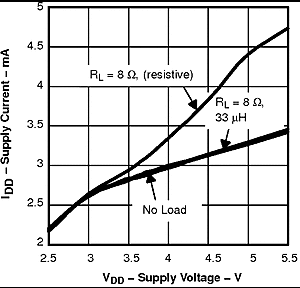Figure 7. Supply Current versus Supply Voltage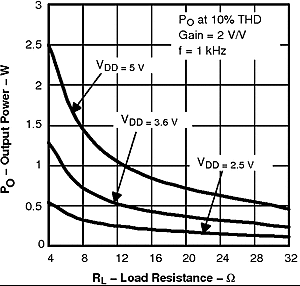Figure 9. Output Power versus Load Resistance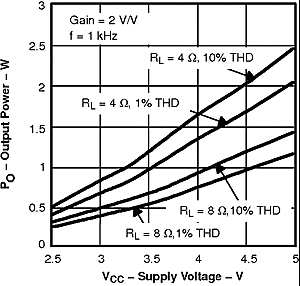Figure 11. Output Power versus Supply Voltage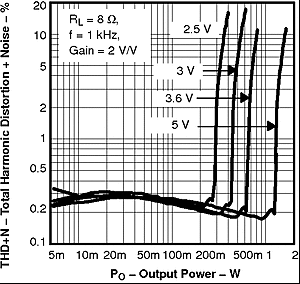Figure 13. Total Harmonic Distortion + Noise versus Output Power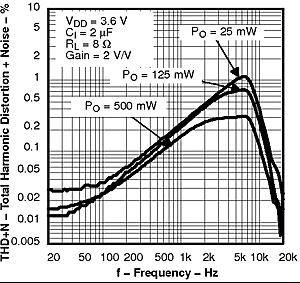Figure 15. Total Harmonic Distortion + Noise versus Frequency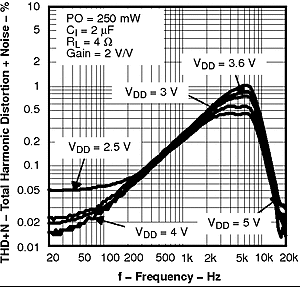Figure 17. Total Harmonic Distortion + Noise versus frequency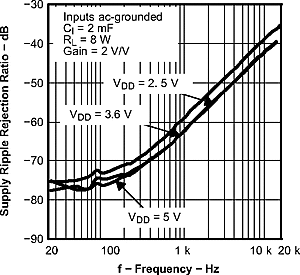Figure 19. Supply Ripple Rejection Ratio versus Frequency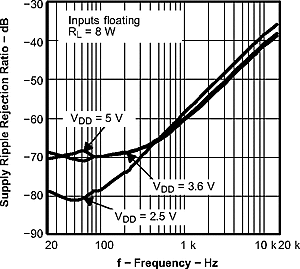Figure 21. Supply Ripple Rejection Ratio versus Frequency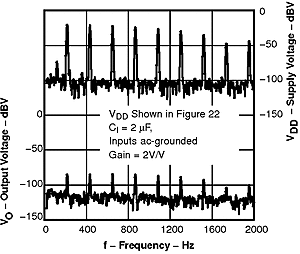Figure 23. GSM Power Supply Rejection versus Frequency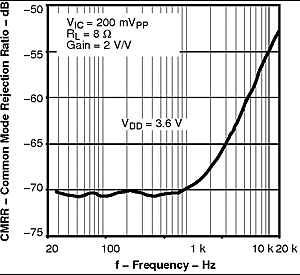Figure 25. Common-Mode Rejection Ratio versus
Frequency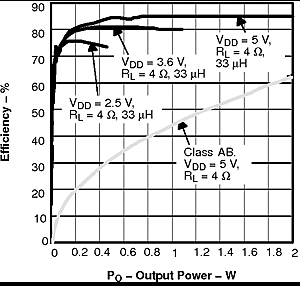Figure 2. Efficiency versus Output Power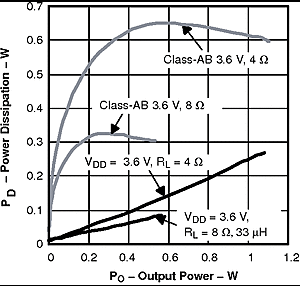Figure 4. Power Dissipation versus Output Power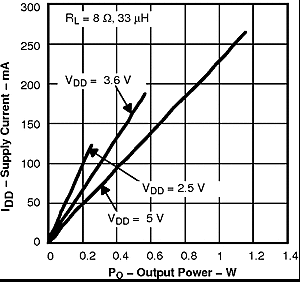Figure 6. Supply Current versus Output Power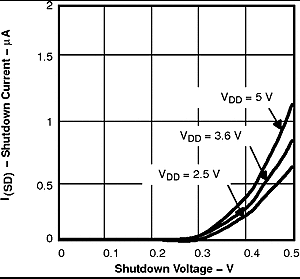Figure 8. Supply Current versus Shutdown Voltage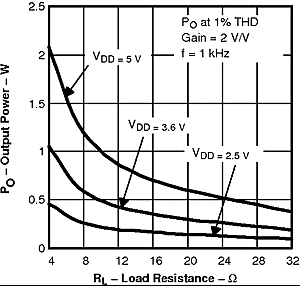Figure 10. Output Power versus Load ResistanceFigure 12. Total Harmonic Distortion + Noise versus Output PowerFigure 14. Total Harmonic Distortion + Noise versus Frequency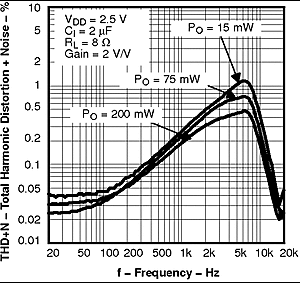Figure 16. Total Harmonic Distortion + Noise versus Frequency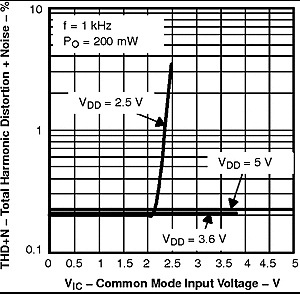Figure 18. Total Harmonic Distortion + Noise versus Common Mode Input Voltage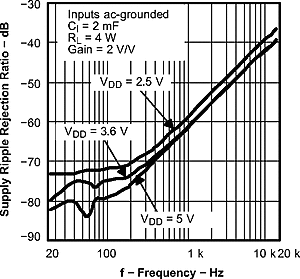Figure 20. Supply Ripple Rejection Ratio versus Frequency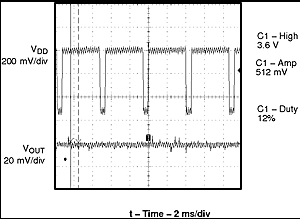Figure 22. GSM Power Supply Rejection versus Time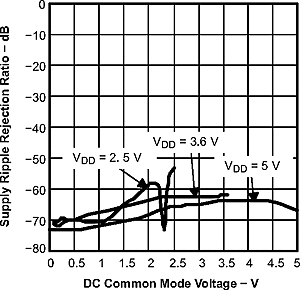Figure 24. Supply Ripple Rejection Ratio versus DC Common Mode Voltage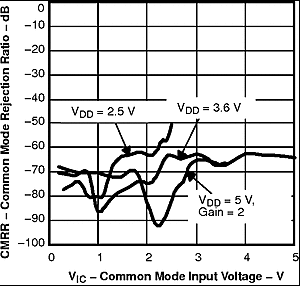Figure 26. Common-Mode Rejection Ratio versus
Common-Mode Input Voltage